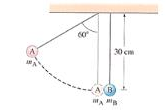# 1. Two balls, of masses m_A=36 g and m_B = 80 g are surrounded as shown in the figure. The...

## Question:

1. Two balls, of masses {eq}m_A=36 g {/eq} and {eq}m_B = 80 g {/eq} are surrounded as shown in the figure. The lighter balls is pulled away to a {eq}60 ^{\circ} {/eq} angle with the vertical and released.a. What is the velocity of the lighter ball before impact?

b. What is the velocity of each ball after the elastic collision?

c. What will be the maximum height of each ball after the elastic collision?

2. An {eq}18 g {/eq} rifle bullet travelling {eq}230 \frac{m}{s} {/eq} buries itself in a {eq}3.6 kg {/eq} pendulum hanging on a {eq}2.6 m {/eq} long string, which makes the pendulum swing upward in an arc. Determine the vertical and horizontal components of the pendulum's displacement?

## Energy conservation

Energy conservation is the principle according to that the total energy at any point remains constant. It changes only the form of energy like potential energy gets converted into the kinetic energy etc.

## Answer and Explanation:

(1)

(a)

The initial height of the lighter ball

{eq}h = L (1- \cos\theta) h = 0.3 (1- \cos\ 60) h = 0.15 \ m {/eq}

Now, from the energy conservation

{eq}m_{a}gh = 0.5m_{a}u^{2} \\ 9.81*0.15 = 0.5*u^{2} \\ u = 1.72 \ m/s {/eq}

Where

• u is the velocity of the lighter ball before impact

(b)

Now, conserving the momentum

{eq}m_{a}u = m_{a}v_{1} +m_{b}v_{2} \\ 36*1.72 = 36v_{1} + 80v_{2} \\ 36v_{1}+80v_{2} = 61.92 \ ----------(1) {/eq}

Now, for the elastic collision

{eq}v_{2} - v_{1} = u \\ v_{2} - v_{1} = 1.72 \ ------------(2) {/eq}

On solving 1 and 2

{eq}v_{1} = -0.65 \ m/s \\ v_{2} = 1.07 \ m/s {/eq}

-ve sign indicates that the motion of the ball A is reversed.

(c)

Now, from the energy conservation

{eq}h_{a} = \dfrac{v_{1}^{2}}{2g} \\ h_{a}= \dfrac{0.65^{2}}{2*9.81} \\ h_{a} = 0.0215 \ m {/eq}

Now, for ball "b"

{eq}h_{b} = \dfrac{v_{2}^{2}}{2g} \\ h_{b}= \dfrac{1.07^{2}}{2*9.81} \\ h_{b} = 0.058\ m {/eq}

(2)

Given

Mass of the bullet(m) = 18 g

Velocity of the bullet (u) = 230 m/s

Mass of the pendulum (M) = 3.6 kg

Length of the string (L) = 2.6 m

Now, applying the conservation of the momentum

{eq}mu = (m+M)V \\ 0.018*230 = (3.6+0.018)V \\ V = 1.144 \ m/s {/eq}

Where

• V is the velocity of the pendulum after the bullet embedded into it

Now, from the energy conservation, we can write

{eq}0.5*(m+M)V^{2} = (m+M)gh \\ 0.5*(0.018+3.6)1.144^{2} = (0.018+3.6)*9.81*h \\ h = 0.067 \ m {/eq}

Where

• h is the height achieved by the pendulum

Now, from the geometry we can write

{eq}L (1- \cos\theta) = h \\ 2.6 (1- \cos\theta) = 0.067 \\ \theta = 13^\circ {/eq}

Now, the horizontal displacement of the pendulum

{eq}X = L\sin\theta \\ X = 2.6 \sin13 \\ X = 0.585 \ m {/eq}

Now, the vertical component of the displacement

{eq}Y = L - L \cos\theta \\ Y = 2.6 - 2.6\cos13 \\ Y = 0.067 \ m {/eq}